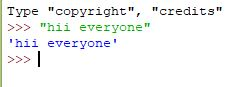Skip to content
Related Articles
Taking input from console in Python
• Difficulty Level : Basic
• Last Updated : 27 Nov, 2020

What is Console in Python? Console (also called Shell) is basically a command line interpreter that takes input from the user i.e one command at a time and interprets it. If it is error free then it runs the command and gives required output otherwise shows the error message. A Python Console looks like this.Here we write command and to execute the command just press enter key and your command will be interpreted.
For coding in Python you must know the basics of the console used in Python.

The primary prompt of the python console is the three greater than symbols

```>>>
```

You are free to write the next command on the shell only when after executing the first command these prompts have appeared. The Python Console accepts command in Python which you write after the prompt.Accepting Input from Console
User enters the values in the Console and that value is then used in the program as it was required.
To take input from the user we make use of a built-in function input().

 `# input``input1 ``=` `input``()`` ` `# output``print``(input1)`

We can also type cast this input to integer, float or string by specifying the input() function inside the type.

1. Typecasting the input to Integer: There might be conditions when you might require integer input from user/Console, the following code takes two input(integer/float) from console and typecasts them to integer then prints the sum.
 `# input``num1 ``=` `int``(``input``())``num2 ``=` `int``(``input``())`` ` `# printing the sum in integer``print``(num1 ``+` `num2)`
2. Typecasting the input to Float: To convert the input to float the following code will work out.
 `# input``num1 ``=` `float``(``input``())``num2 ``=` `float``(``input``())`` ` `# printing the sum in float``print``(num1 ``+` `num2)`
3. Typecasting the input to String: All kind of input can be converted to string type whether they are float or integer. We make use of keyword str for typecasting.
 `# input``string ``=` `str``(``input``())`` ` `# output``print``(string)`

Attention geek! Strengthen your foundations with the Python Programming Foundation Course and learn the basics.

To begin with, your interview preparations Enhance your Data Structures concepts with the Python DS Course. And to begin with your Machine Learning Journey, join the Machine Learning – Basic Level Course

My Personal Notes arrow_drop_up